# 1 Introduction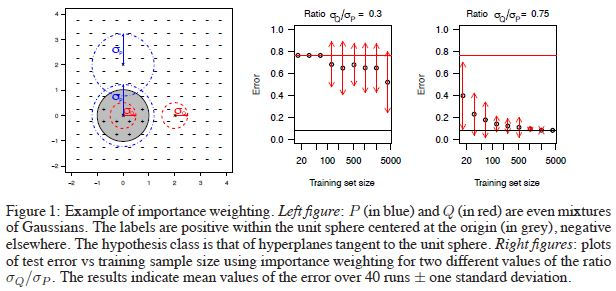# 2 Preliminaries

## 2.1 Renyi Divergences

Renyi熵可以用来衡量两个分布之间的相关性，公式如下：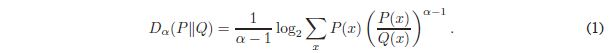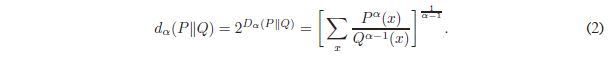## Importance Weight

$P$$Q$的importance weight定义为$w(x) = P(x)/Q(x)$，可以得到如下引理及证明（期望是关于$Q$的）：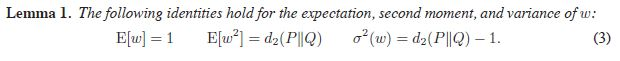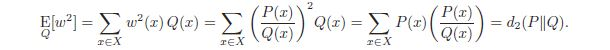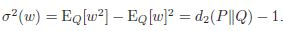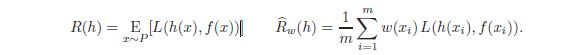$L_h(x)$表示$L(h(x), f(x))$，那么由于非归一化的$w(x)$是无偏的，那么：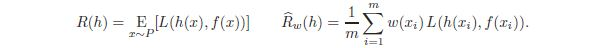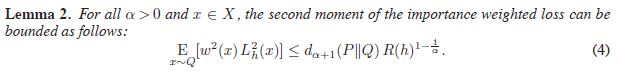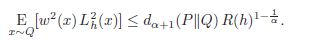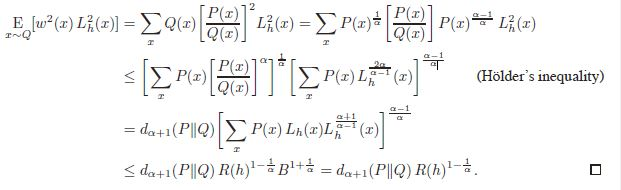# 3 LearningGuarantees-BoundedCase

$w(x)$有界时，令$M=\text{sup}_xw(x)=d_\infin(P||Q)$$d_\alpha(P||Q)$关于$\alpha$单调递增），根据Hoeffding不等式，有以下命题：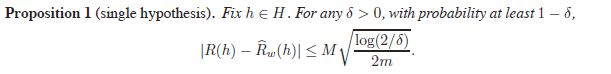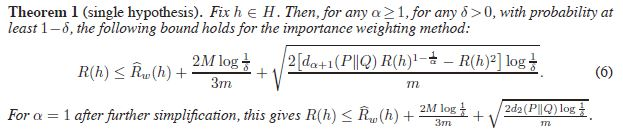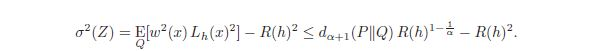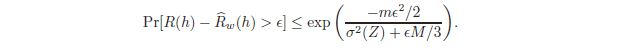$\delta$作为概率上界带入可整理得到下面的不等式以最低概率$1-\delta$成立：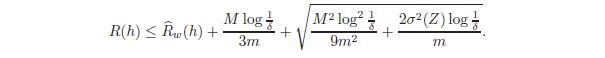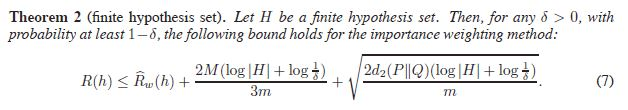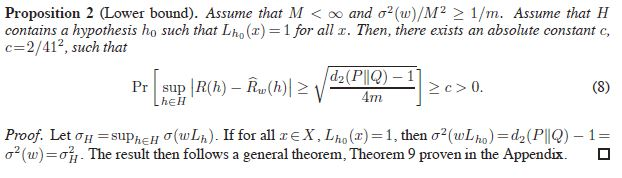# Learning Guarantees - Unbounded Case

$d_\infin(P||Q)<\infin$的条件并不一定有效，下面讨论一些无界的例子。

## 4.1 Examples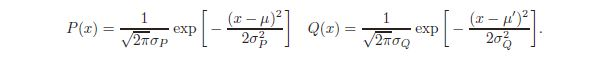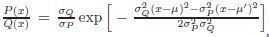importance weights是无界的，2阶的Renyi熵：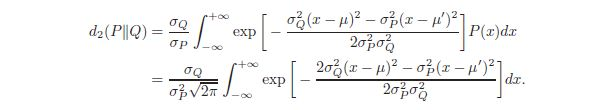## Importance weighting learning bounds - unbounded case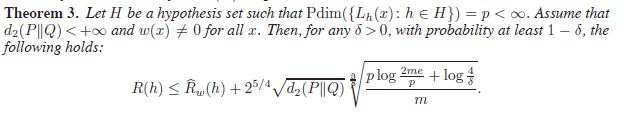# 5 Alternative reweighting algorithms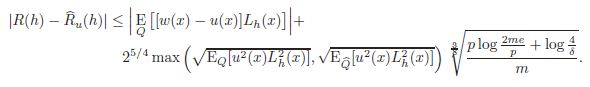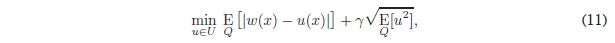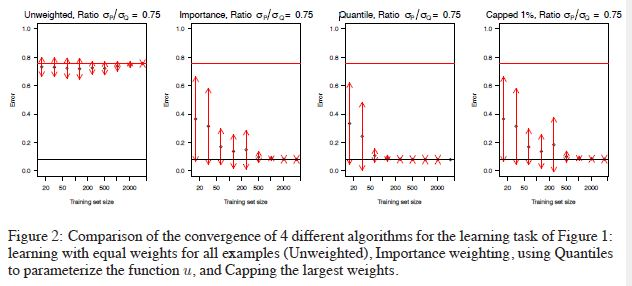# 7 Conclusion

©️2019 CSDN 皮肤主题: 大白 设计师: CSDN官方博客